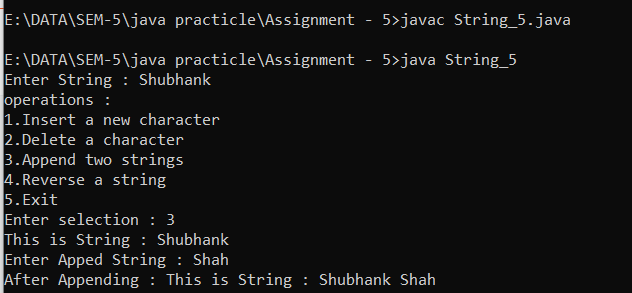# Code

import java.util.Scanner;

interface st{

void Sub();

void len();

void index();

}

Scanner scan = new Scanner(System.in);

String org = "";

this.org = org;

}

public void Sub(){

System.out.print ("Enter SubString : ");

String s1 = scan.next();

int in = org.indexOf (s1);

if(in != -1)

System.out.println ("First occurrence of Substring "+s1+" is found at : " +in);

else

}

public void len(){

int lengt = org.length();

System.out.println (org+" length is : "+lengt);

}

public void index(){

if (org.length() < 5)

System.out.println ("Their are not 5 char in String then replace not possible... ");

else{

System.out.println ("Before changing String : "+org);

System.out.print ("Enter Any Character : ");

char c = scan.next().charAt (0);

if (org.length() == 5)

org = org.substring (0,4) + c;

else

org = org.substring (0,4) + c+ org.substring (4+1);

System.out.println ("After changing String : "+org);

}

}

}

class String_1{

public static void main (String args [] ){

Scanner scan = new Scanner (System.in);

int c = 1, i = 0;

String org = "";

while (c != 5){

if (i == 0){

System.out.print ("Enter String : ");

org = scan.next();

i++;

}

System.out.println ("\n\nSelect From Following Operations \n1.Find a substring\n2.Find a length of the string\n3.A particular character located at index no.5\n4.New String\n5.Exit");

System.out.print ("Enter Selection : ");

c = scan.nextInt();

System.out.println ("\n");

switch (c){

case 1: m1.Sub(); break;

case 2: m1.len(); break;

case 3: m1.index(); break;

case 4: i=0; break;

case 5: System.out.println("Eixting...."); break;

default : System.out.println("Invalid Selection.....");

}

}

}

}

3D Rotation In Computer Graphics

String Operations In Java